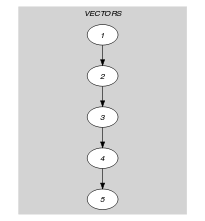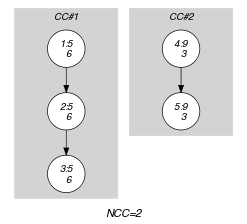## 5.309. ordered_nvector

Origin
Constraint

$\mathrm{𝚘𝚛𝚍𝚎𝚛𝚎𝚍}_\mathrm{𝚗𝚟𝚎𝚌𝚝𝚘𝚛}\left(\mathrm{𝙽𝚅𝙴𝙲},\mathrm{𝚅𝙴𝙲𝚃𝙾𝚁𝚂}\right)$

Synonyms

$\mathrm{𝚘𝚛𝚍𝚎𝚛𝚎𝚍}_\mathrm{𝚗𝚟𝚎𝚌𝚝𝚘𝚛𝚜}$, $\mathrm{𝚘𝚛𝚍𝚎𝚛𝚎𝚍}_\mathrm{𝚗𝚙𝚘𝚒𝚗𝚝}$, $\mathrm{𝚘𝚛𝚍𝚎𝚛𝚎𝚍}_\mathrm{𝚗𝚙𝚘𝚒𝚗𝚝𝚜}$.

Type
 $\mathrm{𝚅𝙴𝙲𝚃𝙾𝚁}$ $\mathrm{𝚌𝚘𝚕𝚕𝚎𝚌𝚝𝚒𝚘𝚗}\left(\mathrm{𝚟𝚊𝚛}-\mathrm{𝚍𝚟𝚊𝚛}\right)$
Arguments
 $\mathrm{𝙽𝚅𝙴𝙲}$ $\mathrm{𝚍𝚟𝚊𝚛}$ $\mathrm{𝚅𝙴𝙲𝚃𝙾𝚁𝚂}$ $\mathrm{𝚌𝚘𝚕𝚕𝚎𝚌𝚝𝚒𝚘𝚗}\left(\mathrm{𝚟𝚎𝚌}-\mathrm{𝚅𝙴𝙲𝚃𝙾𝚁}\right)$
Restrictions
 $|\mathrm{𝚅𝙴𝙲𝚃𝙾𝚁}|\ge 1$ $\mathrm{𝙽𝚅𝙴𝙲}\ge \mathrm{𝚖𝚒𝚗}\left(1,|\mathrm{𝚅𝙴𝙲𝚃𝙾𝚁𝚂}|\right)$ $\mathrm{𝙽𝚅𝙴𝙲}\le |\mathrm{𝚅𝙴𝙲𝚃𝙾𝚁𝚂}|$ $\mathrm{𝚛𝚎𝚚𝚞𝚒𝚛𝚎𝚍}$$\left(\mathrm{𝚅𝙴𝙲𝚃𝙾𝚁𝚂},\mathrm{𝚟𝚎𝚌}\right)$ $\mathrm{𝚜𝚊𝚖𝚎}_\mathrm{𝚜𝚒𝚣𝚎}$$\left(\mathrm{𝚅𝙴𝙲𝚃𝙾𝚁𝚂},\mathrm{𝚟𝚎𝚌}\right)$
Purpose

Enforces the following two conditions:

1. $\mathrm{𝙽𝚅𝙴𝙲}$ is the number of distinct tuples of values assigned to the vectors of the collection $\mathrm{𝚅𝙴𝙲𝚃𝙾𝚁𝚂}$. Two tuples of values $〈{A}_{1},{A}_{2},\cdots ,{A}_{m}〉$ and $〈{B}_{1},{B}_{2},\cdots ,{B}_{m}〉$ are $distinct$ if and only if there exist an integer $i\in \left[1,m\right]$ such that ${A}_{i}\ne {B}_{i}$.

2. For each pair of consecutive vectors ${\mathrm{𝚅𝙴𝙲𝚃𝙾𝚁}}_{i}$ and ${\mathrm{𝚅𝙴𝙲𝚃𝙾𝚁}}_{i+1}$ of the $\mathrm{𝚅𝙴𝙲𝚃𝙾𝚁𝚂}$ collection we have that ${\mathrm{𝚅𝙴𝙲𝚃𝙾𝚁}}_{i}$ is lexicographically less than or equal to ${\mathrm{𝚅𝙴𝙲𝚃𝙾𝚁}}_{i+1}$. Given two vectors, $\stackrel{\to }{X}$ and $\stackrel{\to }{Y}$ of $n$ components, $〈{X}_{0},\cdots ,{X}_{n-1}〉$ and $〈{Y}_{0},\cdots ,{Y}_{n-1}〉$, $\stackrel{\to }{X}$ is lexicographically less than or equal to $\stackrel{\to }{Y}$ if and only if $n=0$ or ${X}_{0}<{Y}_{0}$ or ${X}_{0}={Y}_{0}$ and $〈{X}_{1},\cdots ,{X}_{n-1}〉$ is lexicographically less than or equal to $〈{Y}_{1},\cdots ,{Y}_{n-1}〉$.

Example
$\left(\begin{array}{c}2,〈\begin{array}{c}\mathrm{𝚟𝚎𝚌}-〈5,6〉,\hfill \\ \mathrm{𝚟𝚎𝚌}-〈5,6〉,\hfill \\ \mathrm{𝚟𝚎𝚌}-〈5,6〉,\hfill \\ \mathrm{𝚟𝚎𝚌}-〈9,3〉,\hfill \\ \mathrm{𝚟𝚎𝚌}-〈9,3〉\hfill \end{array}〉\hfill \end{array}\right)$

The $\mathrm{𝚘𝚛𝚍𝚎𝚛𝚎𝚍}_\mathrm{𝚗𝚟𝚎𝚌𝚝𝚘𝚛}$ constraint holds since:

1. Its first argument $\mathrm{𝙽𝚅𝙴𝙲}=2$ is set to the number of distinct tuples of values (i.e., tuples $〈5,6〉$ and $〈9,3〉$) occurring within the collection $\mathrm{𝚅𝙴𝙲𝚃𝙾𝚁𝚂}$.

2. The vectors of the collection $\mathrm{𝚅𝙴𝙲𝚃𝙾𝚁𝚂}$ are sorted in increasing lexicographical order.

Typical
 $|\mathrm{𝚅𝙴𝙲𝚃𝙾𝚁}|>1$ $\mathrm{𝙽𝚅𝙴𝙲}>1$ $\mathrm{𝙽𝚅𝙴𝙲}<|\mathrm{𝚅𝙴𝙲𝚃𝙾𝚁𝚂}|$ $|\mathrm{𝚅𝙴𝙲𝚃𝙾𝚁𝚂}|>1$
Arg. properties
• Functional dependency: $\mathrm{𝙽𝚅𝙴𝙲}$ determined by $\mathrm{𝚅𝙴𝙲𝚃𝙾𝚁𝚂}$.

• Contractible wrt. $\mathrm{𝚅𝙴𝙲𝚃𝙾𝚁𝚂}$ when $\mathrm{𝙽𝚅𝙴𝙲}=1$ and $|\mathrm{𝚅𝙴𝙲𝚃𝙾𝚁𝚂}|>0$.

• Contractible wrt. $\mathrm{𝚅𝙴𝙲𝚃𝙾𝚁𝚂}$ when $\mathrm{𝙽𝚅𝙴𝙲}=|\mathrm{𝚅𝙴𝙲𝚃𝙾𝚁𝚂}|$.

Reformulation

The $\mathrm{𝚘𝚛𝚍𝚎𝚛𝚎𝚍}_\mathrm{𝚗𝚟𝚎𝚌𝚝𝚘𝚛}$ constraint can be reformulated as a conjunction of a $\mathrm{𝚗𝚟𝚎𝚌𝚝𝚘𝚛}$ and a $\mathrm{𝚕𝚎𝚡}_\mathrm{𝚌𝚑𝚊𝚒𝚗}_\mathrm{𝚕𝚎𝚜𝚜𝚎𝚚}$ constraints.

implies: $\mathrm{𝚕𝚎𝚡}_\mathrm{𝚌𝚑𝚊𝚒𝚗}_\mathrm{𝚕𝚎𝚜𝚜𝚎𝚚}$ ($\mathrm{𝙽𝚅𝙴𝙲}$ of constraint $\mathrm{𝚘𝚛𝚍𝚎𝚛𝚎𝚍}_\mathrm{𝚗𝚟𝚎𝚌𝚝𝚘𝚛}$ removed), $\mathrm{𝚗𝚟𝚎𝚌𝚝𝚘𝚛}$, $\mathrm{𝚘𝚛𝚍𝚎𝚛𝚎𝚍}_\mathrm{𝚊𝚝𝚕𝚎𝚊𝚜𝚝}_\mathrm{𝚗𝚟𝚎𝚌𝚝𝚘𝚛}$ ($=\mathrm{𝙽𝚅𝙴𝙲}$ replaced by $\ge \mathrm{𝙽𝚅𝙴𝙲}$), $\mathrm{𝚘𝚛𝚍𝚎𝚛𝚎𝚍}_\mathrm{𝚊𝚝𝚖𝚘𝚜𝚝}_\mathrm{𝚗𝚟𝚎𝚌𝚝𝚘𝚛}$ ($=\mathrm{𝙽𝚅𝙴𝙲}$ replaced by $\le \mathrm{𝙽𝚅𝙴𝙲}$).

Keywords
Arc input(s)

$\mathrm{𝚅𝙴𝙲𝚃𝙾𝚁𝚂}$

Arc generator
$\mathrm{𝑃𝐴𝑇𝐻}$$↦\mathrm{𝚌𝚘𝚕𝚕𝚎𝚌𝚝𝚒𝚘𝚗}\left(\mathrm{𝚟𝚎𝚌𝚝𝚘𝚛𝚜}\mathtt{1},\mathrm{𝚟𝚎𝚌𝚝𝚘𝚛𝚜}\mathtt{2}\right)$

Arc arity
Arc constraint(s)
$\mathrm{𝚕𝚎𝚡}_\mathrm{𝚕𝚎𝚜𝚜𝚎𝚚}$$\left(\mathrm{𝚟𝚎𝚌𝚝𝚘𝚛𝚜}\mathtt{1}.\mathrm{𝚟𝚎𝚌},\mathrm{𝚟𝚎𝚌𝚝𝚘𝚛𝚜}\mathtt{2}.\mathrm{𝚟𝚎𝚌}\right)$
Graph property(ies)
$\mathrm{𝐍𝐀𝐑𝐂}$$=|\mathrm{𝚅𝙴𝙲𝚃𝙾𝚁𝚂}|-1$

Arc input(s)

$\mathrm{𝚅𝙴𝙲𝚃𝙾𝚁𝚂}$

Arc generator
$\mathrm{𝑃𝐴𝑇𝐻}$$↦\mathrm{𝚌𝚘𝚕𝚕𝚎𝚌𝚝𝚒𝚘𝚗}\left(\mathrm{𝚟𝚎𝚌𝚝𝚘𝚛𝚜}\mathtt{1},\mathrm{𝚟𝚎𝚌𝚝𝚘𝚛𝚜}\mathtt{2}\right)$

Arc arity
Arc constraint(s)
$\mathrm{𝚕𝚎𝚡}_\mathrm{𝚕𝚎𝚜𝚜}$$\left(\mathrm{𝚟𝚎𝚌𝚝𝚘𝚛𝚜}\mathtt{1}.\mathrm{𝚟𝚎𝚌},\mathrm{𝚟𝚎𝚌𝚝𝚘𝚛𝚜}\mathtt{2}.\mathrm{𝚟𝚎𝚌}\right)$
Graph property(ies)
$\mathrm{𝐍𝐂𝐂}$$=\mathrm{𝙽𝚅𝙴𝙲}$

Graph model

Parts (A) and (B) of Figure 5.309.1 respectively show the initial and final graph of the second graph constraint associated with the Example slot. Since we use the $\mathrm{𝐍𝐂𝐂}$ graph property in this second graph constraint, we show the different connected components of the final graph. Each strongly connected component corresponds to a tuple of values that is assigned to some vectors of the $\mathrm{𝚅𝙴𝙲𝚃𝙾𝚁𝚂}$ collection. The 2 following tuple of values $〈5,6〉$ and $〈9,3〉$ are used by the vectors of the $\mathrm{𝚅𝙴𝙲𝚃𝙾𝚁𝚂}$ collection.

##### Figure 5.309.1. Initial and final graph of the $\mathrm{𝚘𝚛𝚍𝚎𝚛𝚎𝚍}_\mathrm{𝚗𝚟𝚎𝚌𝚝𝚘𝚛}$ constraint(a) (b)